# http://www.planetb.ca/syntax-highlight-word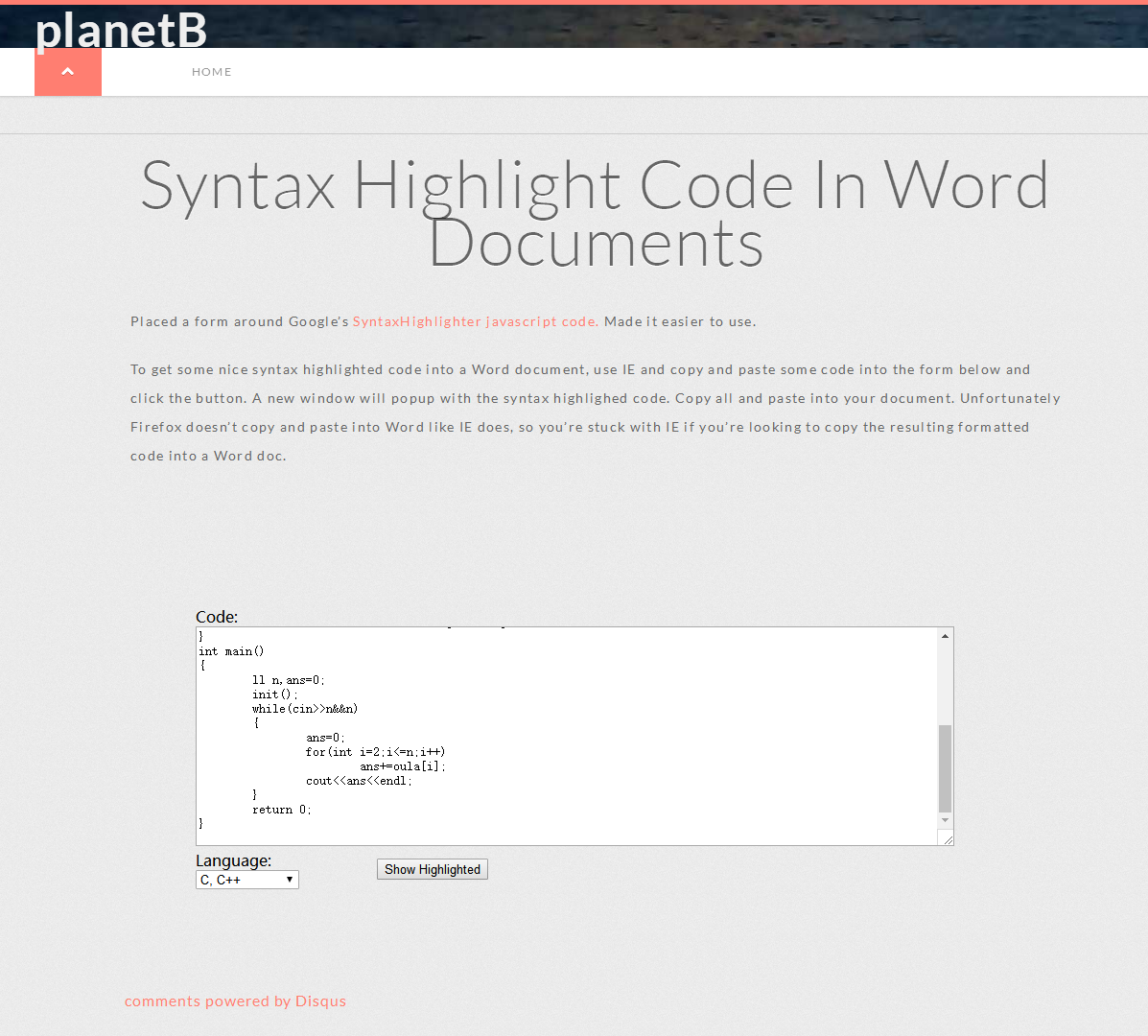生成的代码窗体复制粘贴到word上就好啦。

## 原【模拟】NewBuildingforSIS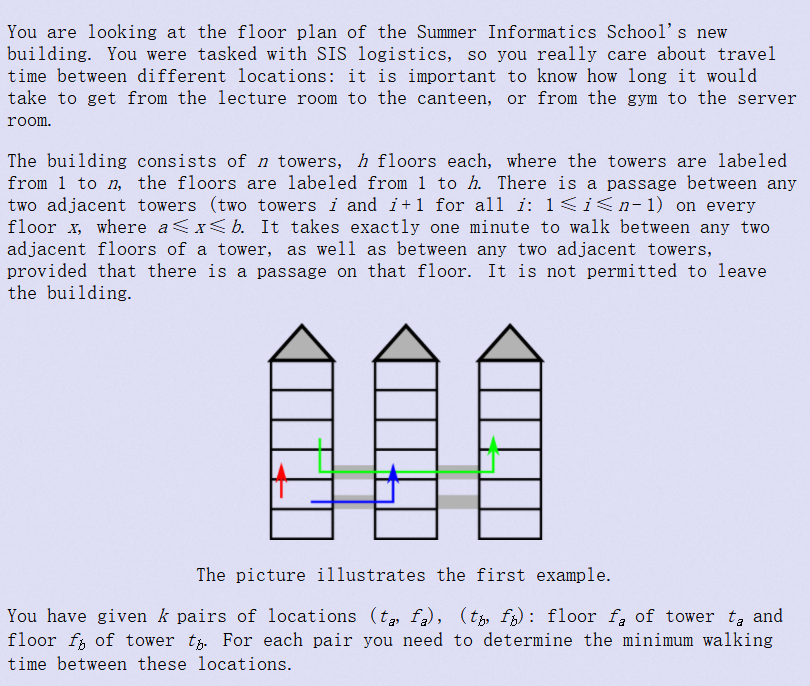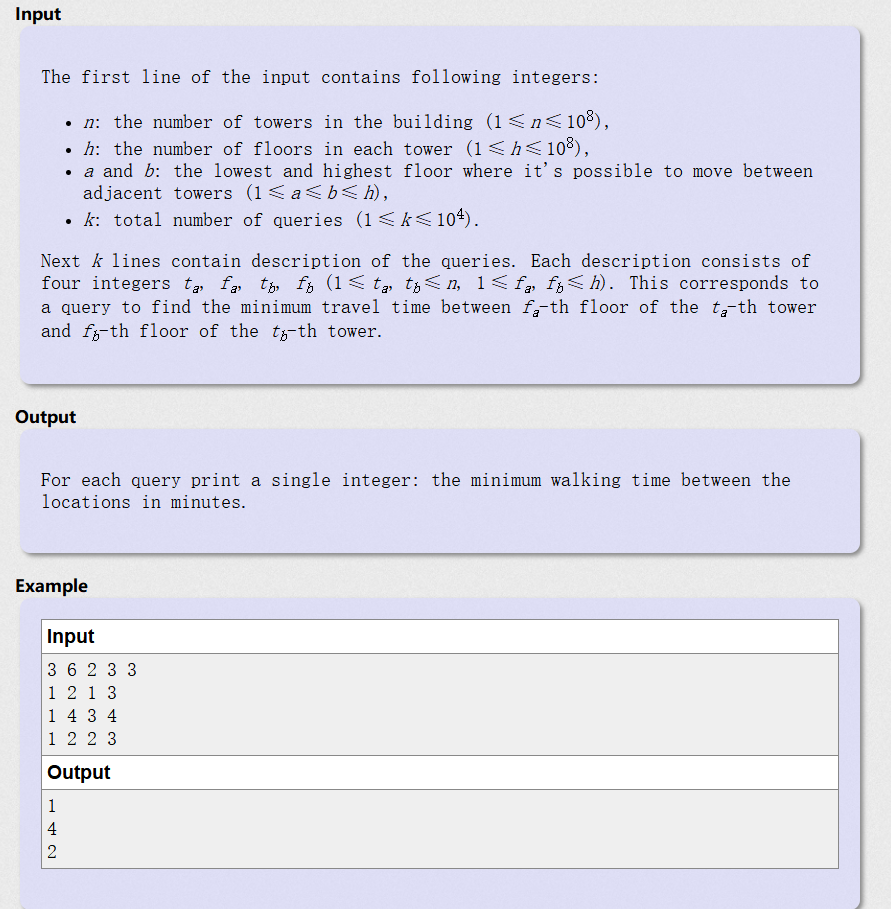# 题意：给你n个相邻的建筑（从左到右编号1到n），每个建筑有h层，每个建筑的a层到b层中任意一层c层可以花一秒通过连廊直接到隔壁建筑的c层。上下楼1层需要1秒。求q次查询(建筑a,层a)到(建筑b,层b)的最短时间。

#include <iostream>
#include <cmath>
#include <cstdio>
using namespace std;
int main()
{
int n,h,a,b,k;
scanf("%d%d%d%d%d",&n,&h,&a,&b,&k);
while(k--)
{
int ta,fa,tb,fb;long long ans=0;
scanf("%d%d%d%d",&ta,&fa,&tb,&fb);
if(ta==tb)
ans+=abs(fb-fa);//同楼层
if(ta!=tb)
{
ans+=abs(tb-ta);//计算横向移动时间
if(fa>=a&&fa<=b)
{
ans+=abs(fb-fa);//如果都处于连廊直接通过的楼层，附加纵向距离
}
else
{
int temp1=0,temp2=0;
temp1+=abs(fa-a);//temp1代表如果从最下层连廊走
temp1+=abs(fb-a);
temp2+=abs(fa-b);//temp1代表如果从最上层连廊走
temp2+=abs(fb-b);
ans+=min(temp1,temp2);//取最短距离
}
}
cout<<ans<<endl;
}
return 0;
}

# Codeforces Round 48 (Rated for Div. 2)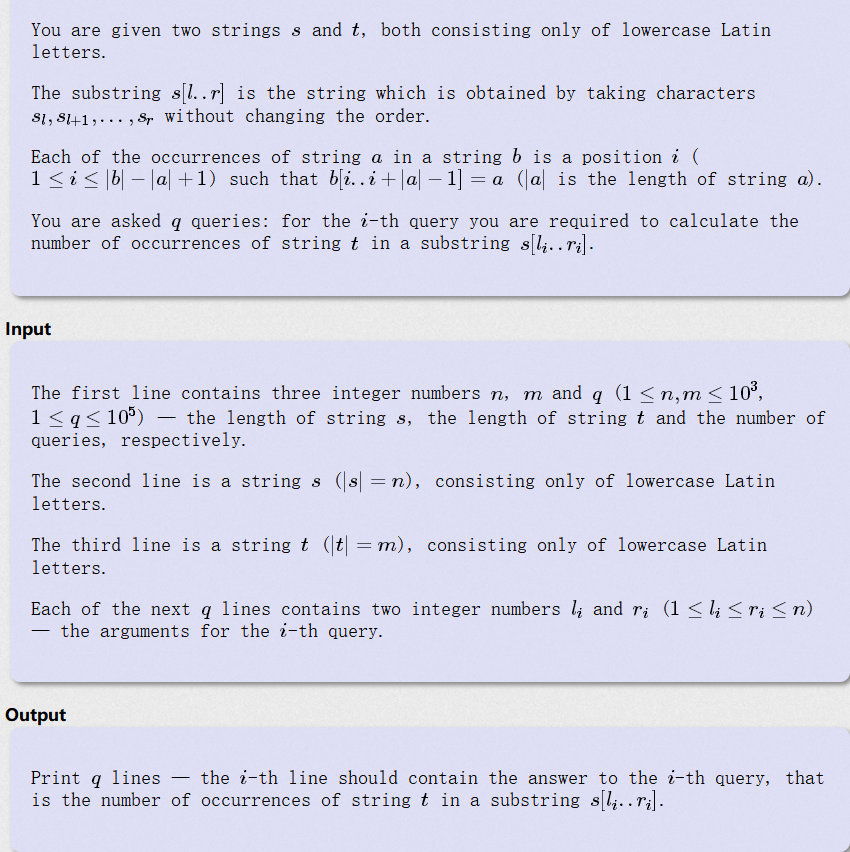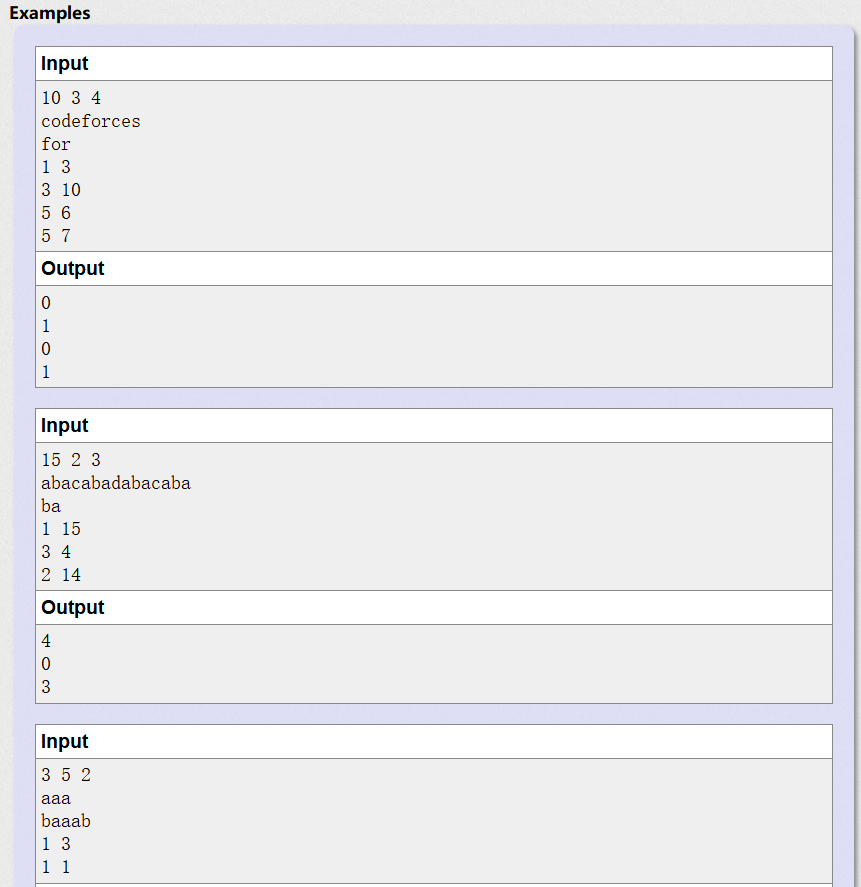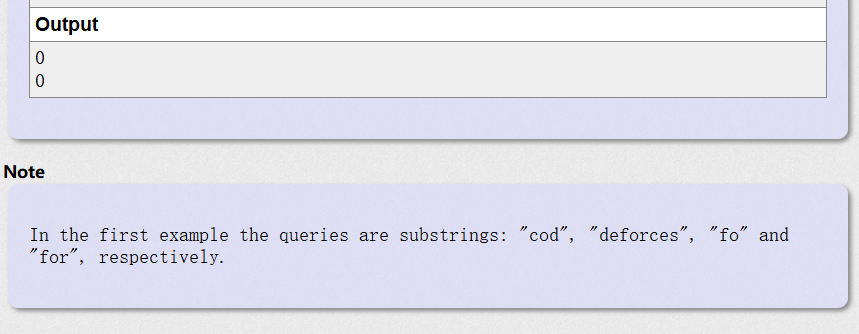# 大意：输入字符串s和t、各自的长度和q个询问，询问由第l到r个字母构成的s的子串中，包含t的个数

#include <iostream>
#include <string>
#include <cstdio>
using namespace std;
int n,m,w,sum=0;
string s,t;
int main()
{
int n,m,q;
scanf("%d%d%d",&n,&m,&q);//s长度,n长度，q次数
s.resize(1200);
t.resize(1200);
scanf("%s%s",&s,&t);//s串，t串
int l,r,ans=0;
while(q--)
{
ans=0;
scanf("%d%d",&l,&r);
if(r-l+1>=m)
{
for(int i=l-1;i+m-1<r;i++)
{
string temp=s.substr(i,m);
if(s.substr(i,m)==t.substr(0,m)) ans++;
}
}
printf("%d\n",ans);

}
}


# 后来采用了类似打表的思想过了。

#include<iostream>
#include<cstdio>
#include<string>
using namespace std;
int a;
int main()
{
int n,m,k,q,l,r,ans;
string s,t;
cin>>n>>m>>q;
cin>>s>>t;
for(int i=0; i<=n-m; i++)
if(s.substr(i,m)==t)
a[i]=1;
while(q--)
{
ans=0;
cin>>r>>l;
for(int i=r-1; i<=l-m; i++)
if(a[i])
ans++;
cout<<ans<<endl;
}
return 0;
}


# 别人家的题解：

#include<cstdio>
#include<cstring>
#include<string>
#include<iostream>
#include<algorithm>
using namespace std;
int main()
{
int n,m,q,i,j,l,r,len;
int counts;
int vis;
string s1,s2;
cin>>n>>m>>q;
cin>>s1>>s2;
len=s2.size();
memset(vis,0,sizeof(vis));
string::size_type pos=0;
while((pos=s1.find(s2,pos))!=string::npos)
{
vis[pos+1]=pos+1;
pos++;
}
for(i=1;i<=q;i++)
{
counts=0;
scanf("%d%d",&l,&r);
for(j=l;j<=r;j++)
{
if(vis[j]!=0&&vis[j]+len-1<=r)
{
counts++;
}
}
printf("%d\n",counts);
}
return 0;
}

# 还有前缀数组的大佬：

#include <bits/stdc++.h>
using namespace std;
int n,m,q;
string s,t;
int l,r;
int hay,shay;
int main()
{
cin>>n>>m>>q;
cin>>s>>t;
s=" "+s;
t=" "+t;
for(int i=1;i<=n-m+1;i++)
{
hay[i]=1;
for(int j=0;j<m;j++)
{
if(s[i+j]!=t[1+j])
{
hay[i]=0;
break;
}
}
shay[i]=shay[i-1]+hay[i];
}

while(q--)
{
cin>>l>>r;
r=r-m+1;
if(r<l) cout<<"0"<<endl;
else cout<<shay[r]-shay[l-1]<<endl;
}
return 0;
}

## SDAU训练日志第一篇排序算法（上）（2018年1月29日）

SDAU训练日志第一篇排序算法（上）（2018年1月29日）

经过了在山东农业大学一学期的学习，我们迎来了耿霞老师开设C++程序设计课程的期末考试，在程序设计题的第一题给我们出了一道这样的题：

（(✺ω✺)）给你一组数据（1<n<1000），数据中可能有重复，你的任务是完成去重，并按照从小到大顺序输出数据。（NOIP2006）

1.先去重再排序输出   2. 先排序再去重输出（重复的都在一起）

#include<bits/stdc++.h>
using namespace std;
const int MAXN=1000;
int main()
{
bool flag[MAXN+1];
//因为数组下标是少1的，我们多加一个元素表示maxn
memset(flag,0,sizeof(flag));
//初始化该桶
int n=0,temp=0;
cin>>n;
for(int i=1;i<=n;i++)
{
cin>>temp;
flag[temp]=true;
//标记该桶
}
for(int i=1;i<=1000;i++)
if(flag[i])
cout<<i<<' ';
return 0;
}

#include<bits/stdc++.h>
using namespace std;
int main()
{
int shuzu;
memset(shuzu,0,sizeof(shuzu));
int n=0;
cin>>n;
for(int i=0;i<n;i++)
cin>>shuzu[i];
sort(shuzu,shuzu+n);
cout<<shuzu<<' ';
for(int i=1;i<n;i++)
{
if(shuzu[i]!=shuzu[i-1])//不重复就输出
cout<<shuzu[i]<<' ';
}
return 0;
}

#include<iostream>
using namespace std;
const int MAXN=1000;
int main()
{
int flag[MAXN+1];
//因为数组下标是少1的，我们多加一个元素表示maxn
memset(flag,0,sizeof(flag));
//初始化该桶
int n=0,temp=0;
cin>>n;
for(int i=1;i<=MAXN;i++)
{
cin>>temp;
flag[temp]++;
}
for(int i=1;i<=MAXN;i++)
for(int j=1;j<=flag[i];j++)//划重点
cout<<i<<' ';
return 0;
}

1. 只能对整数进行排序
2. 以空间的方式纵使降低了时间复杂度，然而空间的浪费问题严重
3. 无法处理有复杂要求的排序问题

void bubble_sort(int arr[], int len) {
int i, j;
for (i = 0; i < len - 1; i++)
for (j = 0; j < len - 1 - i; j++)
if (arr[j] > arr[j + 1])
swap(arr[j], arr[j + 1]);
}  

void bubble_sort(int arr[], int len) {
int i, j;
bool flag;
for (i = 0; i < len - 1 && flag!=0; i++)
{
flag=0;
for (j = 0; j < len - 1 - i; j++)
if (arr[j] > arr[j + 1])
{
swap(arr[j], arr[j + 1]);
flag = 1;
}
}
}  

鸡尾酒排序又叫定向冒泡排序，搅拌排序、来回排序等，是冒泡排序的一种变形。此算法与冒泡排序的不同处在于排序时是以双向在序列中进行排序。

void cocktail_sort(int arr[], int len) {
int j, left = 0, right = len - 1;
while (left < right) {
for (j = left; j < right; j++)
if (arr[j] > arr[j + 1])
swap(arr[j], arr[j + 1]);
right--;
for (j = right; j > left; j--)
if (arr[j - 1] > arr[j])
swap(arr[j - 1], arr[j]);
left++;
}
}  

void SelectSort(int arr[],int n)
{
for(int i = 0; i <n - 2; ++i)
{
int k = i;
for(int j = i + 1; j < n - 1; ++j)
{
//找到最小的数的下标
if(v[j] < v[k])
k = j;
}
if(k != i)
{
swap(v[k],v[i]);
}
}
}

void SelectSort(int arr[],int n)
{
int left = 0;
int right = n - 1;
int min = left;//用来存储最小值的下标
int max = left;//用来存储最大值的下标
while(left <= right)
{
min = left;
max = left;
for(int i = left; i <= right; i++)
{
if(arr[i]<arr[min])
min = i;
if(arr[i] > arr[max])
max = i;
}
swap(arr[left],arr[min]);
if(left == max)
max = min;
swap(arr[right],arr[max]);
left++,right++;
}
}

• 桶排序，以空间换时间，对于处理取值范围小的领域占优势（比如学生成绩），但处理取值大的数据或者有较复杂要求的排序题就弱鸡了，限制较高
• 选择和冒泡，占用空间比桶排序要小，可是时间复杂度超级高，当排序数据量高的时候会慢出翔。